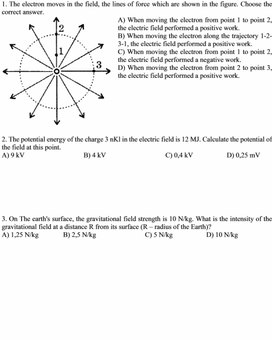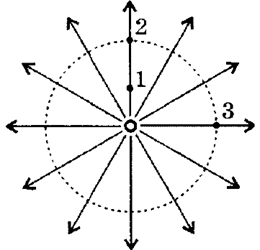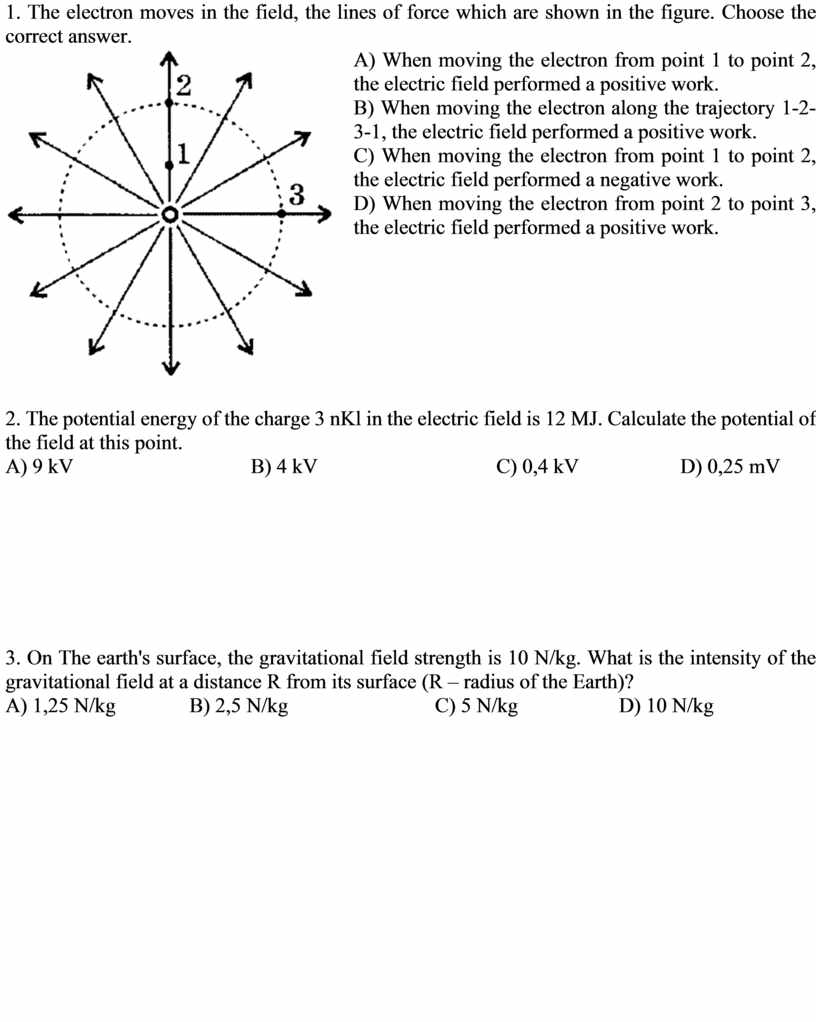# ФО

Оценка 4.8
docx
07.05.2020ФО.docx

1. The electron moves in the field, the lines of force which are shown in the figure. Choose the correct answer.А) When moving the electron from point 1 to point 2, the electric field performed a positive work.

В) When moving the electron along the trajectory 1-2-3-1, the electric field performed a positive work.

С) When moving the electron from point 1 to point 2, the electric field performed a negative work.

D) When moving the electron from point 2 to point 3, the electric field performed a positive work.

2. The potential energy of the charge 3 nKl in the electric field is 12 MJ. Calculate the potential of the field at this point.

А) 9 kV                                  В) 4 kV                                  С) 0,4 kV                   D) 0,25 mV

3. On The earth's surface, the gravitational field strength is 10 N/kg. What is the intensity of the gravitational field at a distance R from its surface (R – radius of the Earth)?

А) 1,25 N/kg              В) 2,5 N/kg                             С) 5 N/kg                    D) 10 N/kg

## The electron moves in the field, the lines of force which are shown in the figureСкачать файл